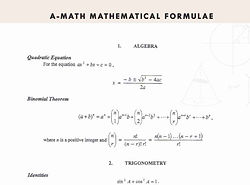Having math notes available for students is crucial to their success, as most math classes include a heavy amount of math problems that require practice. These math notes includes everything from the basics of fractions and decimals, all the way to more complex math topics such as matrices and permutations! Start with the table of contents below, or check out each individual math note by clicking above. Good luck!

A-MATH NOTES SUMMARYFormula List A-math formulas for Sec School students